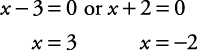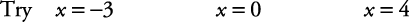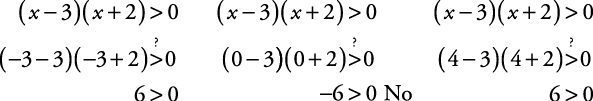• Solve the inequality as though it were an equation.

The real solutions to the equation become boundary points for the solution to the inequality.

• Make the boundary points solid circles if the original inequality includes equality; otherwise, make the boundary points open circles.

• Select points from each of the regions created by the boundary points. Replace these “test points” in the original inequality.

• If a test point satisfies the original inequality, then the region that contains that test point is part of the solution.

• Represent the solution in graphic form and in solution set form.

##### Example 1

Solve ( x – 3)( x + 2) > 0.

Solve ( x – 3)( x + 2) = 0. By the zero product property,Make the boundary points. Here, the boundary points are open circles because the original inequality does not include equality (see Figure 1).

Select points from the different regions created (see Figure 2).See whether the test points satisfy the original inequality.Since x = –3 satisfies the original inequality, the region x < –2 is part of the solution. Since x = 0 does not satisfy the original inequality, the region –2 < x < 3 is not part of the solution. Since x = 4 satisfies the original inequality, the region x > 3 is part of the solution.

Represent the solution in graphic form and in solution set form. The graphic form is shown in Figure 3.

The solution set form is { x| x < –2 or x > 3}.